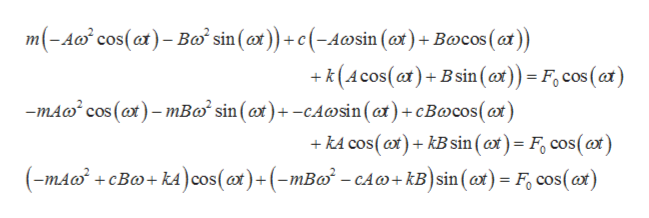# Take mx′′+cx′+kx=F0cos(ωt).mx″+cx′+kx=F0cos⁡(ωt). Fix c>0,c>0, k>0,k>0, and F0>0.F0>0. Consider the function C(ω).C(ω). For what values of mm (solve in terms of c,c, k,k, and F0F0) will there be no practical resonance (that is, for what values of mm is there no maximum of C(ω)C(ω) for ω>0ω>0)?

Question
13 views

Take mx′′+cx′+kx=F0cos(ωt).mx″+cx′+kx=F0cos⁡(ωt). Fix c>0,c>0, k>0,k>0, and F0>0.F0>0. Consider the function C(ω).C(ω). For what values of mm (solve in terms of c,c, k,k, and F0F0) will there be no practical resonance (that is, for what values of mm is there no maximum of C(ω)C(ω) for ω>0ω>0)?

check_circle

Step 1

First find the particular solution for the differential equation-

Step 2

Auxiliary solution is

Step 3

Substitute the obtained solution...help_outlineImage Transcriptionclosem(-A cos(at)-Ba sin(at))+c(-Awsin (ax)+Bcos (at)) k(Acos(at)+Bsin (ox)) = F cos (ar) mA cos (ax)-mBø° sin(@x)+ -cAwsin (at)+ cBwcos() kA cos (at)kB sin (ct)= F cos(at) (mACBkA cos(a)+(-mBa -cA+kB)sin (ax)= F cos(ax) fullscreen

### Want to see the full answer?

See Solution

#### Want to see this answer and more?

Solutions are written by subject experts who are available 24/7. Questions are typically answered within 1 hour.*

See Solution
*Response times may vary by subject and question.
Tagged in

### Math